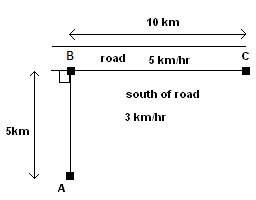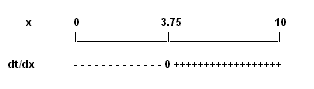# Use Derivatives to solve problems: Distance-time Optimization

A problem to minimize (optimization) the time taken to walk from one point to another is presented. An analytical method, using derivatives and other calculus concepts and theorems, is developed in order to find an analytical solution to the problem.

## Problem

You decide to walk from point A (see figure below) to point C. To the south of the road through BC, the terrain is difficult and you can only walk at 3 km/hr. However, along the road BC you can walk at 5 km/hr. The distance from point A to the road is 5 km. The distance from B to C is 10 km. What path you have to follow in order to arrive at point C in the shortest ( minimum ) time possible?Solution to the Problem

We now look at a solution using derivatives and other calculus concepts. Let distance BP be equal to x. Let us find a formula for the distances AP and PC. Using Pythagorean theorm, we can write:
distance AP = √(5 2 + x 2)

distance PC = 10 - x

We now find time t
1 to walk distance AP.(time = distance / speed).
t 1 = distance AP / 3 = √(5 2 + x 2) / 3

Time t
2 to walk distance PC is given by
t 2 = distance PC / 5 = (10 - x) / 5

The total time t is found by adding t
1 and t 2 .
t = √(5
2 + x 2 ) / 3 + (10 - x) / 5
we might consider the domain of function t as being all values of x in the closed interval [0 , 10]. For values of x such that point P is to the left of B or to the right of c, time t will increase.
To find the value of x that gives t minimum, we need to find the first derivative dt/dx (t is a functions of x).
dt/dx = (x/3) / √(5
2 + x 2 ) - 1/5
If t has a minimum value, it happens at x such that dt/dx = 0.
(x/3) / √(5
2 + x 2 ) - 1/5 = 0
Solve the above for x. Rewrite the equation as follows.
5x = 3√(5
2 + x 2 )
Square both sides.
25x
2 = 9(5 2 + x 2 )
Group like terms and simplify
16x
2 = 225
Solve for x (x >0 )
x = √(225/16) = 3.75 km.
dt/dx has one zero. The table of sign of the first derivative dt/dx is shown below.The first derivative dt/dx is negative for x < 3.75, equal to zero at x = 3.75 and positive for x >3.75. Also the values of t at x = 0 and x = 10 (the endpoints of the domain of t) are respectively 3.6 hrs and 3.7 hrs. The value of t at
x = 3.75 is equal to 3.3 hrs and its is the smallest. The answer to our problem is that one has to walk to point P such BP = 3.75 km then procced along the road to C in order to get there in the shortest possible time.
Exercises

1 - Solve the same problem as above but with the following values.solution to the above exercise
x = 6.26 km (rounded to 2 decimal places).
More references on
calculus problems